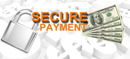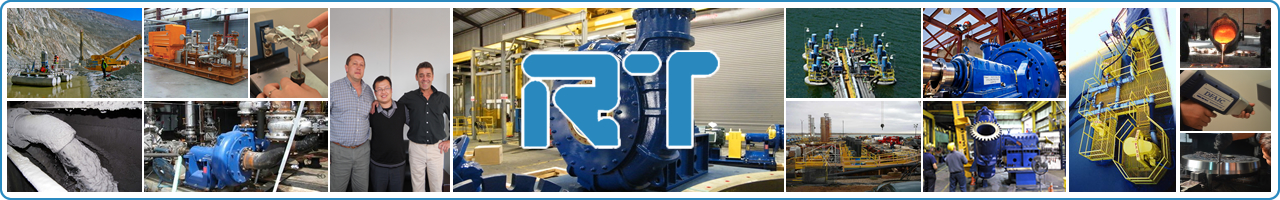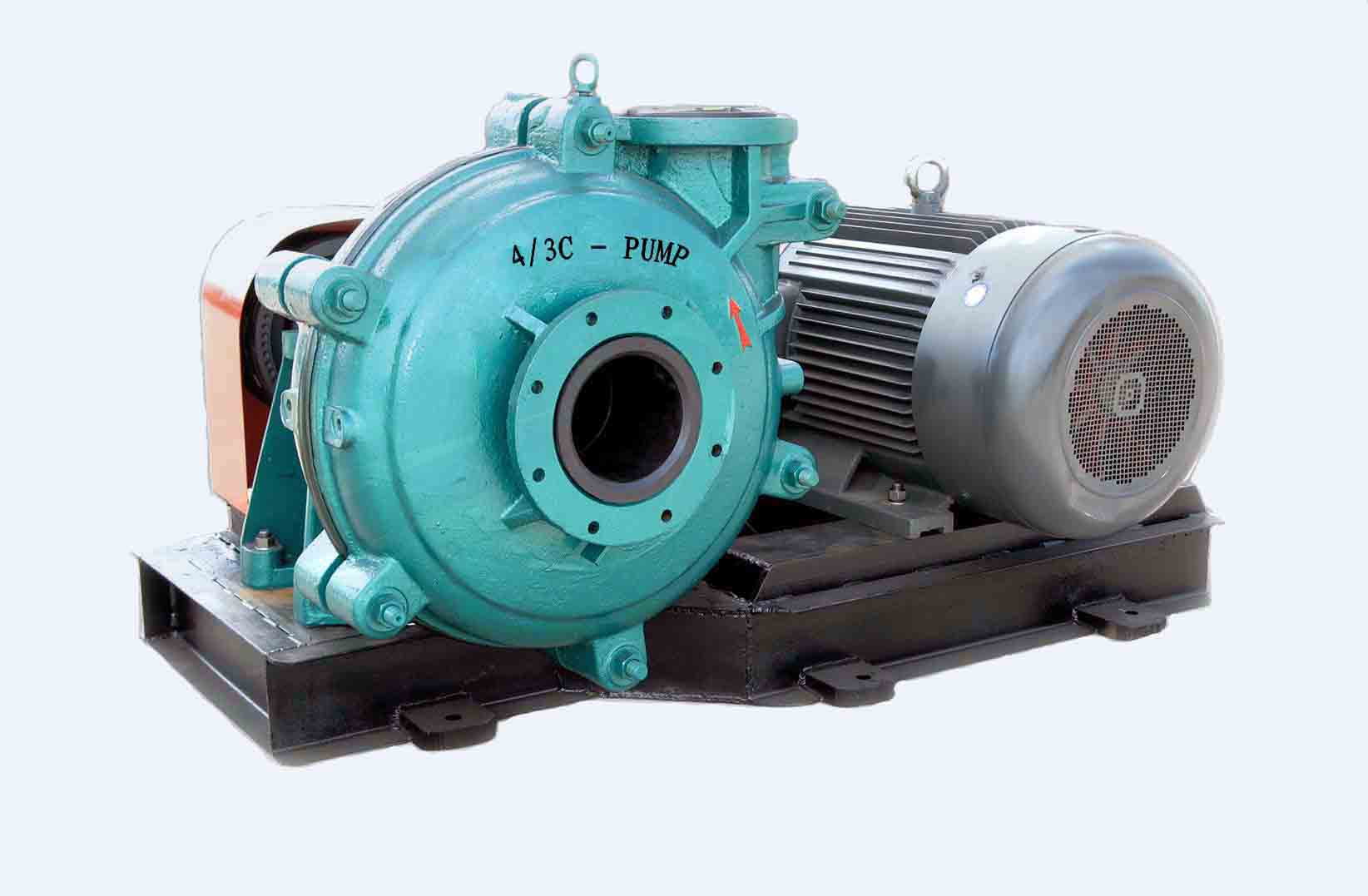# 渣浆泵轴功率是什么，如何计算？## 渣浆泵轴功率计算方法-通用计算公式

(1)离心泵

P=2.73HQ/η,

P=ρgQH/1000η（kw),其中的ρ=1000Kg/m3,g=9.8 kg/s*s

=Kg/m3*m3/h*m*9.8牛顿/Kg

=9.8牛顿*m/3600秒

=牛顿*m/367秒

=瓦/367

Ne≤22 K=1.25

22<Ne≤55 K=1.15

100<Ne K无限接近1.1

(2)渣浆泵轴功率计算公式

N=H*Q*A*g/(n*3600)

(3)泵的效率及其计算公式

Pe=ρg QH (W) 或Pe=γQH/1000 （KW）

ρ：泵输送液体的密度（kg/m3）

γ：泵输送液体的重度 γ=ρg （N/ m3）

g：重力加速度（m/s2）